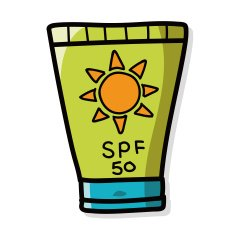(Not Double Science - Chemistry GCSE Only)

1. What is the size range of nanoparticles?
• A.   0.1 nm to 1 nm
• B.   1 nm to 10 nm
• C.   1 nm to 100 nm
• D.   1 nm to 1000 nm
2. What is the approximate size of an atom?
• A.   100 nm
• B.   10 nm
• C.   1 nm
• D.   0.1 nm
3. What is the surface area of a cube with sides of 2 nm?
• A.   4 nm2
• B.   8 nm2
• C.   16 nm2
• D.   24 nm2
4. What is the volume of a cube with sides of 5 nm?
• A.   25 nm3
• B.   75 nm3
• C.   125 nm3
• D.   150 nm3
5. What is the surface area to volume ratio of a cube with sides 10 nm in length?
• A.   0.1
• B.   0.4
• C.   0.6
• D.   0.8
6. How does the surface area to volume ratio change if the sides of a cube decrease by a factor of 10?
• A.   decreases by a factor of 2
• B.   decreases by a factor of 10
• C.   increases by a factor of 2
• D.   increases by a factor of 10
7. What is a bulk material?
• A.   A substance in the form of powder, lumps or sheets
• B.   A substance in the form of nanoparticles
• C.   A large amount of nanoparticle material
• D.   A substance with a high molecular mass

8. Nanoparticles of zinc oxide can be used in sunscreen. They are better than powdered zinc oxide because they ...• A.   are less toxic
• B.   are invisible on the skin
• C.   don’t block ultraviolet radiation
• D.   tend to clump together
9. There are possible risks associated with the use of nanoparticles due to their small size.

Which of the following is NOT a possible risk of using nanoparticles?

• A.   They may be inhaled
• B.   Toxic substances may bind to them
• C.   They may be absorbed into cells
• D.   They may be more efficient catalysts than the same substance in bulk

10. Which of the following statements about nanoparticles are true?

 I. Nanoparticles have a large surface area to volume ratio II. Nanoparticles may have different properties than the same material in bulk III. Nanoparticles can be seen under an optical microscope

• A.   I and II only
• B.   I and III only
• C.   II and III only
• D.   I, II and III